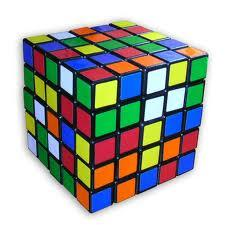# Tereza

The cube has area of base 256 mm2.

Calculate the edge length, volume and area of its surface.

Correct result:

a =  16 mm
V =  4096 mm3
S2 =  1536 mm2

#### Solution:

$S=256 \ \text{mm}^2 \ \\ S=a^2=a \cdot \ a \ \\ a=\sqrt{ S }=\sqrt{ 256 }=16 \ \text{mm}$
$V=S \cdot \ a=256 \cdot \ 16=4096 \ \text{mm}^3$
$S_{2}=6 \cdot \ S=6 \cdot \ 256=1536 \ \text{mm}^2$We would be very happy if you find an error in the example, spelling mistakes, or inaccuracies, and please send it to us. We thank you!

Showing 1 comment:Math student
Nice but boring#### You need to know the following knowledge to solve this word math problem:

We encourage you to watch this tutorial video on this math problem:

## Next similar math problems:

• Calculate 3Calculate the cube volume whose edge is 3x-1,3x-1,3x-1
• Cube surfce2volumeCalculate the volume of the cube if its surface is 150 cm2.
• Cuboid to cubeA cuboid with dimensions of 9 cm, 6 cm, and 4 cm has the same volume as a cube. Calculate the surface of this cube.
• Length of the edgeFind the length of the edge of a cube that has a cm2 surface and a volume in cm3 expressed by the same number.
• Magnified cubeIf the lengths of the edges of the cube are extended by 5 cm, its volume will increase by 485 cm3. Determine the surface of both the original and the magnified cube.
• EightEight small Christmas balls with a radius of 1 cm have the same volume as one large Christmas ball. What has a bigger surface: eight small balls, or one big ball?
• Alien shipThe alien ship has the shape of a sphere with a radius of r = 3000m, and its crew needs the ship to carry the collected research material in a cuboid box with a square base. Determine the length of the base and (and height h) so that the box has the large
• Cube diagonalsCalculate the length of the side and the diagonals of the cube with a volume of 27 cm3.
• Body diagonalCalculate the cube volume, whose body diagonal size is 75 dm. Draw a picture and highlight the body diagonal.
• Cube into cylinderIf we dip a wooden cube into a barrel with a 40cm radius, the water will rise 10 cm. What is the size of the cube edge?
• Cuboid and ratioFind the dimensions of a cuboid having a volume of 810 cm3 if the lengths of its edges coming from the same vertex are in ratio 2: 3: 5
• Wall thicknessThe hollow metal ball has an outside diameter of 40 cm. Determine the wall thickness if the weight is 25 kg and the metal density is 8.45 g/cm3.
• The volume 2The volume of a cube is 27 cubic meters. Find the height of the cube.
• Right circular coneThe volume of a right circular cone is 5 liters. Calculate the volume of the two parts into which the cone is divided by a plane parallel to the base, one-third of the way down from the vertex to the base.
• The ballThe ball has a radius of 2m. What percentage of the surface and volume is another sphere whose radius is 20% larger?
• Spherical segmentCalculate the volume of a spherical segment 18 cm high. The diameter of the lower base is 80 cm, the upper base 60 cm.
• Volume of ballFind the volume of a volleyball that has a radius of 4 1/2 decimeters. Use 22/7 for π